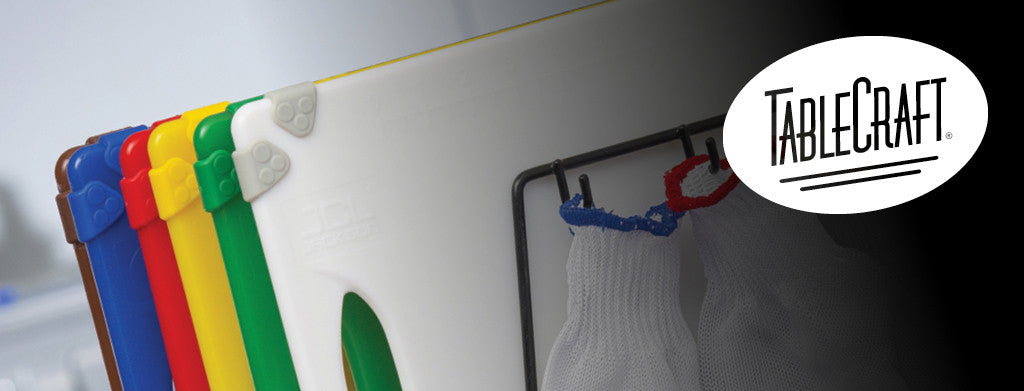Shopping Cart

### Refine

View all

#### Price# Tablecraft£13.18£3.74 each (ex VAT)£6.36 each (ex VAT)£8.38 each (ex VAT)£9.10 each (ex VAT)£8.90 each (ex VAT)£10.48 each (ex VAT)£13.06 each (ex VAT)£3.68 each (ex VAT)£5.02 each (ex VAT)£5.62 each (ex VAT)£4.24 each (ex VAT)£5.22 each (ex VAT)£6.12 each (ex VAT)£3.74 each (ex VAT)£6.36 each (ex VAT)£8.38 each (ex VAT)£9.10 each (ex VAT)£8.90 each (ex VAT)£10.48 each (ex VAT)£13.06 each (ex VAT)£3.68 each (ex VAT)£5.02 each (ex VAT)£5.62 each (ex VAT)£4.24 each (ex VAT)£5.22 each (ex VAT)£6.12 each (ex VAT)£3.74 each (ex VAT)£6.36 each (ex VAT)£8.38 each (ex VAT)£10.02 each (ex VAT)£8.90 each (ex VAT)£10.48 each (ex VAT)£13.06 each (ex VAT)£3.68 each (ex VAT)£5.02 each (ex VAT)£5.62 each (ex VAT)£4.24 each (ex VAT)£5.22 each (ex VAT)£6.12 each (ex VAT)£3.74 each (ex VAT)£6.36 each (ex VAT)£8.38 each (ex VAT)£9.10 each (ex VAT)£8.90 each (ex VAT)£10.48 each (ex VAT)£13.06 each (ex VAT)£3.68 each (ex VAT)